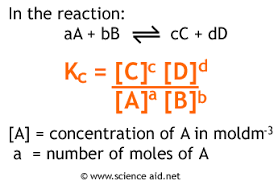# Edexcel Chemistry - Topic 10: Equilibrium I

?
• Created by: Ryan C-S
• Created on: 06-04-18 22:30

## Dynamic Equilibrium

• Dynamic equilibrium occurs when forward and backward reactions are occurring at equal rates
• The concentrations of reactants and products stays constant and the reaction is continuous
• All reversible reactions reach a position of dynamic equilibrium
• The position of equilibrium describes the composition of the equilibrium mixture:
If the position of equilibrium favours the reactants (towards the left) then the mixture will contain mostly reactants
If the position of equilibrium favour the products (towards the right) then the mixture will contain mostly products
• A reversible reaction has an exothermic and an endothermic reaction.
• N2 + 3H2 ⇌ 2NH3
1 of 8

## Le Chatelier's Principle

• Le Chatelier's Principle states that if an external condition is changed the equilibrium will shift to oppose the change and try to reverse it
• Le Chatelier's Principle is used to work out how changing external conditions affect the position of equilibrium

Equilibrium is affected by:

• Temperature
• Concentration
• Pressure
2 of 8

## Effect of Temperature on Equilibrium

• If temperature is increased the equilibrium will shift to oppose this and move in the endothermic direction to try and reduce the temperature by absorbing heat
• If temperature is decreased the equilibrium will shift to oppose this and move in the exothermic direction to try and increase the temperature by giving out heat
• NH2 + 3H2  2NH3 (Exothermic)
• If the temperature is increased the equilibrium will shift to oppose this and move in the endothermic, backwards direction to try to decrease temperature. The position of equilibrium will shift towards the left, giving a lower yield of ammonia

Low temperatures may give a higher yield of a product but will also result in slow rates of reaction. Often a compromise temperature is used that gives a reasonable yield and rate

3 of 8

## Effect of Pressure on Equilibrium

• Increasing pressure will cause the equilibrium to shift towards the side with fewer moles of gas to oppose the change and thereby reduce the pressure
• Decreasing pressure will cause the equilibrium to shift towards the side with more moles of gas to oppose the change and thereby increase the pressure
• If the number of moles of gas is the same on both sides of the equation then changing pressure will have no effect on the position of the equilibrium but will cause a faster rate of reaction
• CO(g) + 2 H2(g)  CH3OH(g)
• If pressure is increased, the equilibrium will shift to oppose this and move towards the side with fewer moles of gas to try and reduce the pressure. The position of the equilibrium will shift towards the right as there are 3 moles of gas on the left but only 1 mole of gas on the right, giving a higher yield of methanol

Increasing pressure may give a higher yield of product and a faster rate but is expensive to produce high pressures and requires expensive technical equipment

4 of 8

## Effect of Concentration on Equilibrium

• I2 + 2OH-  I- + IO- + H2O
brown       ⇌     colourless
• Increasing the concentration ofOH- ions causes the equilibrium to shift to oppose this and move in the forward direction to remove OH- ions. The position of equilibrium will shift towards the right, giving a higher yield of I- and IO- (The colour would change from brown to colourless)
• Increasing the concentration of H+ ions causes the equilibrium to shift to oppose this and move in the backwards direction to increase OH- ions. The position of equilibrium will shift towards the left, giving a higher yield of I2 and OH- (The colour would change from colourless to brown)
5 of 8

## Effect of Catalysts on Equilibrium

• A catalyst has no effect on the position of equilibrium, but it will speed up the rate at which the equilibrium is achieved
• It doesn't effect the position of equilibrium because it speeds up the rates of both the forward and backward reactions by the same amount
• Catalysts are used in industries to reduce the activation energies of a reaction and thereby reduce the overall cost of the reaction making it more economically viable
6 of 8

## The Haber Process

N2 + 3H2 2NH3

T = 450C, P = 200-1000atm catalyst = Iron

• Low temperatures give good yield but slow rate: compromise temp used
• High pressure gives a good yield and high rate: too high a pressure would lead to too high energy costs for pumps to produce the pressure
• Ammonia produced from the Haber Process is used in the manufacturing of fertilisers, explosives and household cleaners
7 of 8

## Equilibrium Constant

• The equilibrium constant can be calculated by the equation below• The larger the Kc, the greater the amount of products.
• If Kc is small, the equilibrium favours the reactants
• Kc only changes with temperature*.
*Pressure, Concentration and Catalysts have no effect on Kc
8 of 8